Goniometric form

Determine goniometric form of a complex number.

Result

Mod z =  5.83
Arg z =  20.06 °

Solution:Leave us a comment of example and its solution (i.e. if it is still somewhat unclear...):Be the first to comment!To solve this verbal math problem are needed these knowledge from mathematics:

Try our complex numbers calculator. Most natural application of trigonometry and trigonometric functions is a calculation of the triangles. Common and less common calculations of different types of triangles offers our triangle calculator. Word trigonometry comes from Greek and literally means triangle calculation. Pythagorean theorem is the base for the right triangle calculator.

Next similar examples:Given that P = (5, 8) and Q = (6, 9), find the component form and magnitude of vector PQ.
2. De Moivre's formula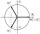There are two distinct complex numbers z such that z3 is equal to 1 and z is not equal 1. Calculate the sum of these two numbers.
3. ABS CNCalculate the absolute value of complex number -15-29i.
4. Moivre 2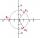Find the cube roots of 125(cos 288° + i sin 288°).
5. Complex number coordinatesWhich coordinates show the location of -2+3i
6. Im>0?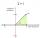Is -10i a positive number?
7. Imaginary numbersFind two imaginary numbers whose sum is a real number. How are the two imaginary numbers related? What is its sum?
8. Linear imaginary equationGiven that ? "this is z star" Find the value of the complex number z.
9. Log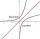Calculate value of expression log |3 +7i +5i2| .
10. ReciprocalCalculate reciprocal of z=0.8-1.8i:
11. Is complexAre these numbers 2i, 4i, 2i + 1, 8i, 2i + 3, 4 + 7i, 8i, 8i + 4, 5i, 6i, 3i complex?
12. Theorem proveWe want to prove the sentence: If the natural number n is divisible by six, then n is divisible by three. From what assumption we started?
13. If theIf the tangent of an angle of a right angled triangle is 0.8. Then its longest side is. .. .
14. PyramidPyramid has a base a = 5cm and height in v = 8 cm. a) calculate angle between plane ABV and base plane b) calculate angle between opposite side edges.On a straight stretch of road is marked 12 percent drop. What angle makes the direction of the road with the horizontal plane?Find the reference angle of each angle: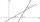Calculate the angle between these two lines: ? ?